## Rational Functions

### Learning Outcomes

• Use arrow notation to describe end behavior of rational functions.
• Solve applied problems involving rational functions.
• Find the domains of rational functions.
• Identify vertical and horizontal asymptotes of graphs of rational functions.
• Graph rational functions.

Suppose we know that the cost of making a product is dependent on the number of items, $x$, produced. This is given by the equation $C\left(x\right)=15,000x - 0.1{x}^{2}+1000$. If we want to know the average cost for producing $x$ items, we would divide the cost function by the number of items, $x$.

The average cost function, which yields the average cost per item for $x$ items produced, is

$f\left(x\right)=\dfrac{15,000x - 0.1{x}^{2}+1000}{x}$

Many other application problems require finding an average value in a similar way, giving us variables in the denominator. Written without a variable in the denominator, this function will contain a negative integer power.

In the last few sections, we have worked with polynomial functions, which are functions with non-negative integers for exponents. In this section, we explore rational functions, which have variables in the denominator.

## Characteristics of Rational Functions

We have seen the graphs of the basic reciprocal function and the squared reciprocal function from our study of toolkit functions. Examine these graphs and notice some of their features.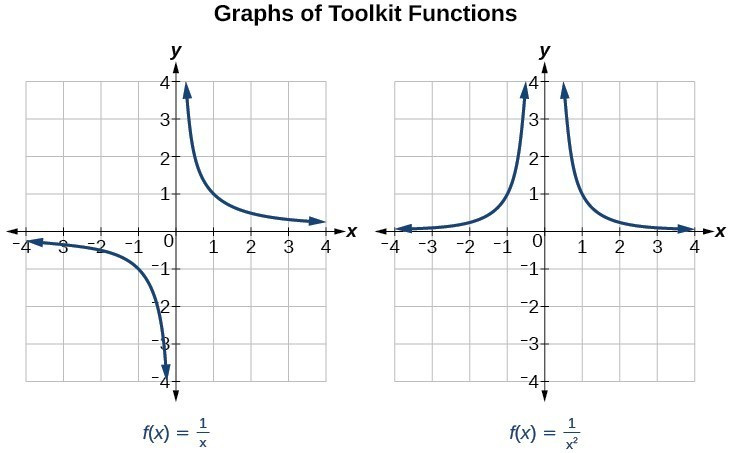Several things are apparent if we examine the graph of $f\left(x\right)=\dfrac{1}{x}$.

1. On the left branch of the graph, the curve approaches the $x$-axis $\left(y=0\right) \text{ as } x\to -\infty$.
2. As the graph approaches $x=0$ from the left, the curve drops, but as we approach zero from the right, the curve rises.
3. Finally, on the right branch of the graph, the curves approaches the $x$axis $\left(y=0\right) \text{ as } x\to \infty$.

To summarize, we use arrow notation to show that $x$ or $f\left(x\right)$ is approaching a particular value.

Arrow Notation
Symbol Meaning
$x\to {a}^{-}$ $x$ approaches $a$ from the left ($x<a$ but close to $a$)
$x\to {a}^{+}$ $x$ approaches $a$ from the right ($x<a$ but close to $a$)
$x\to \infty$ $x$ approaches infinity ($x$ increases without bound)
$x\to -\infty$ $x$ approaches negative infinity ($x$ decreases without bound)
$f\left(x\right)\to \infty$ the output approaches infinity (the output increases without bound)
$f\left(x\right)\to -\infty$ the output approaches negative infinity (the output decreases without bound)
$f\left(x\right)\to a$ the output approaches $a$

## Local Behavior of $f\left(x\right)=\frac{1}{x}$

Let’s begin by looking at the reciprocal function, $f\left(x\right)=\frac{1}{x}$. We cannot divide by zero, which means the function is undefined at $x=0$; so zero is not in the domain. As the input values approach zero from the left side (becoming very small, negative values), the function values decrease without bound (in other words, they approach negative infinity). We can see this behavior in the table below.

 $x$ –0.1 –0.01 –0.001 –0.0001 $f\left(x\right)=\frac{1}{x}$ –10 –100 –1000 –10,000

We write in arrow notation

$\text{as }x\to {0}^{-},f\left(x\right)\to -\infty$

As the input values approach zero from the right side (becoming very small, positive values), the function values increase without bound (approaching infinity). We can see this behavior in the table below.

 $x$ 0.1 0.01 0.001 0.0001 $f\left(x\right)=\frac{1}{x}$ 10 100 1000 10,000

We write in arrow notation

$\text{As }x\to {0}^{+}, f\left(x\right)\to \infty$.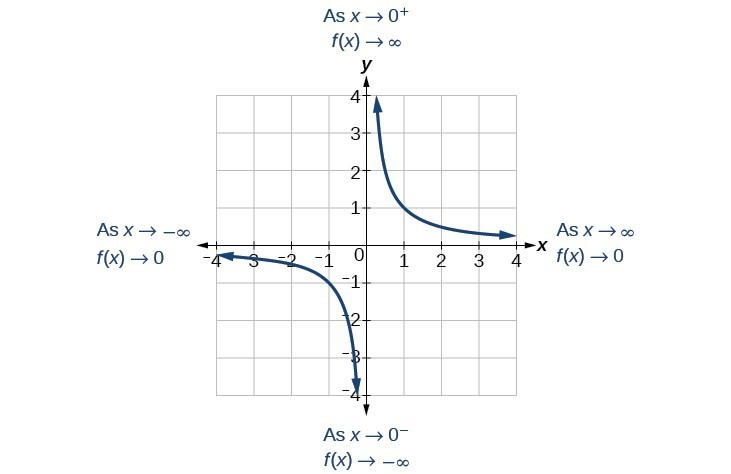This behavior creates a vertical asymptote, which is a vertical line that the graph approaches but never crosses. In this case, the graph is approaching the vertical line $x=0$ as the input becomes close to zero.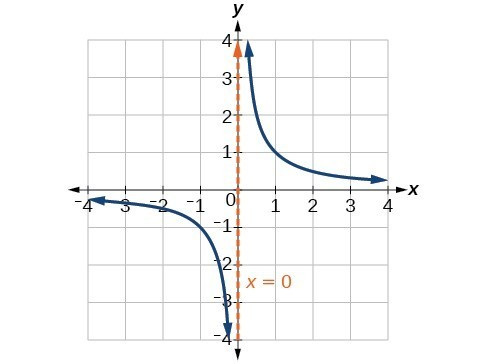### A General Note: Vertical Asymptote

A vertical asymptote of a graph is a vertical line $x=a$ where the graph tends toward positive or negative infinity as the inputs approach $a$. We write

$\text{As }x\to a,f\left(x\right)\to \infty , \text{or as }x\to a,f\left(x\right)\to -\infty$.

## End Behavior of $f\left(x\right)=\frac{1}{x}$

As the values of $x$ approach infinity, the function values approach 0. As the values of $x$ approach negative infinity, the function values approach 0. Symbolically, using arrow notation

$\text{As }x\to \infty ,f\left(x\right)\to 0,\text{and as }x\to -\infty ,f\left(x\right)\to 0$.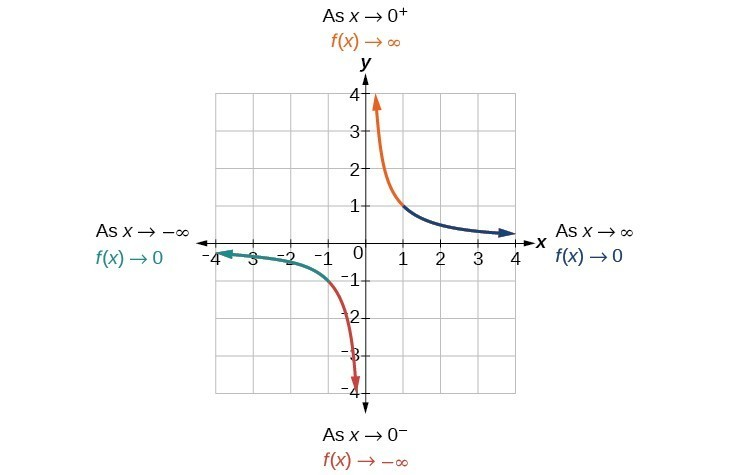Based on this overall behavior and the graph, we can see that the function approaches 0 but never actually reaches 0; it seems to level off as the inputs become large. This behavior creates a horizontal asymptote, a horizontal line that the graph approaches as the input increases or decreases without bound. In this case, the graph is approaching the horizontal line $y=0$.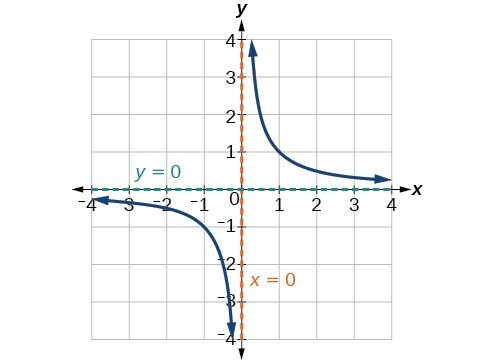### A General Note: Horizontal Asymptote

A horizontal asymptote of a graph is a horizontal line $y=b$ where the graph approaches the line as the inputs increase or decrease without bound. We write

$\text{As }x\to \infty \text{ or }x\to -\infty ,\text{ }f\left(x\right)\to b$.

### Example: Using Arrow Notation

Use arrow notation to describe the end behavior and local behavior of the function below.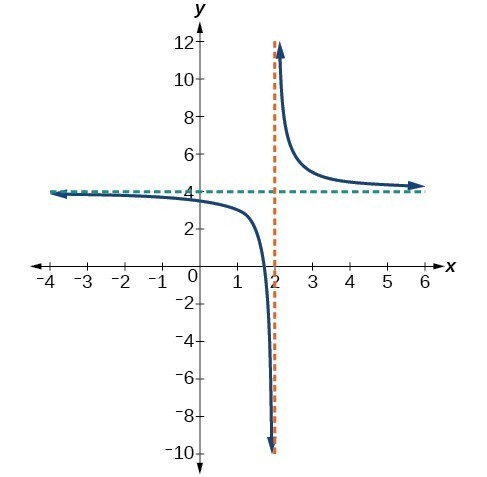### Try It

Use arrow notation to describe the end behavior and local behavior for the reciprocal squared function.

### Example: Using Transformations to Graph a Rational Function

Sketch a graph of the reciprocal function shifted two units to the left and up three units. Identify the horizontal and vertical asymptotes of the graph, if any.

### Try It

Sketch the graph, and find the horizontal and vertical asymptotes of the reciprocal squared function that has been shifted right 3 units and down 4 units.

## A Mixing Problem

In the previous example, we shifted a toolkit function in a way that resulted in the function $f\left(x\right)=\dfrac{3x+7}{x+2}$. This is an example of a rational function. A rational function is a function that can be written as the quotient of two polynomial functions. Many real-world problems require us to find the ratio of two polynomial functions. Problems involving rates and concentrations often involve rational functions.

### A General Note: Rational Function

A rational function is a function that can be written as the quotient of two polynomial functions $P\left(x\right) \text{and} Q\left(x\right)$.

$f\left(x\right)=\dfrac{P\left(x\right)}{Q\left(x\right)}=\dfrac{{a}_{p}{x}^{p}+{a}_{p - 1}{x}^{p - 1}+…+{a}_{1}x+{a}_{0}}{{b}_{q}{x}^{q}+{b}_{q - 1}{x}^{q - 1}+…+{b}_{1}x+{b}_{0}},Q\left(x\right)\ne 0$

### Example: Solving an Applied Problem Involving a Rational Function

A large mixing tank currently contains 100 gallons of water into which 5 pounds of sugar have been mixed. A tap will open pouring 10 gallons per minute of water into the tank at the same time sugar is poured into the tank at a rate of 1 pound per minute. Find the concentration (pounds per gallon) of sugar in the tank after 12 minutes. Is that a greater concentration than at the beginning?

### Try It

There are 1,200 freshmen and 1,500 sophomores at a prep rally at noon. After 12 p.m., 20 freshmen arrive at the rally every five minutes while 15 sophomores leave the rally. Find the ratio of freshmen to sophomores at 1 p.m.

## Domain and Its Effect on Vertical Asymptotes

A vertical asymptote represents a value at which a rational function is undefined, so that value is not in the domain of the function. A reciprocal function cannot have values in its domain that cause the denominator to equal zero. In general, to find the domain of a rational function, we need to determine which inputs would cause division by zero.

### A General Note: Domain of a Rational Function

The domain of a rational function includes all real numbers except those that cause the denominator to equal zero.

### How To: Given a rational function, find the domain.

1. Set the denominator equal to zero.
2. Solve to find the $x$-values that cause the denominator to equal zero.
3. The domain is all real numbers except those found in Step 2.

### Example: Finding the Domain of a Rational Function

Find the domain of $f\left(x\right)=\dfrac{x+3}{{x}^{2}-9}$.

### Try It

Find the domain of $f\left(x\right)=\dfrac{4x}{5\left(x - 1\right)\left(x - 5\right)}$.

By looking at the graph of a rational function, we can investigate its local behavior and easily see whether there are asymptotes. We may even be able to approximate their location. Even without the graph, however, we can still determine whether a given rational function has any asymptotes, and calculate their location.

Watch the following video to see more examples of finding the domain of a rational function.

## Vertical Asymptotes

The vertical asymptotes of a rational function may be found by examining the factors of the denominator that are not common to the factors in the numerator. Vertical asymptotes occur at the zeros of such factors.

### How To: Given a rational function, identify any vertical asymptotes of its graph.

1. Factor the numerator and denominator.
2. Note any restrictions in the domain of the function.
3. Reduce the expression by canceling common factors in the numerator and the denominator.
4. Note any values that cause the denominator to be zero in this simplified version. These are where the vertical asymptotes occur.
5. Note any restrictions in the domain where asymptotes do not occur. These are removable discontinuities.

### Example: Identifying Vertical Asymptotes

Find the vertical asymptotes of the graph of $k\left(x\right)=\dfrac{5+2{x}^{2}}{2-x-{x}^{2}}$.

## Removable Discontinuities

Occasionally, a graph will contain a hole: a single point where the graph is not defined, indicated by an open circle. We call such a hole a removable discontinuity.

For example the function $f\left(x\right)=\dfrac{{x}^{2}-1}{{x}^{2}-2x - 3}$ may be re-written by factoring the numerator and the denominator.

$f\left(x\right)=\dfrac{\left(x+1\right)\left(x - 1\right)}{\left(x+1\right)\left(x - 3\right)}$

Notice that $x+1$ is a common factor to the numerator and the denominator. The zero of this factor, $x=-1$, is the location of the removable discontinuity. Notice also that $x - 3$ is not a factor in both the numerator and denominator. The zero of this factor, $x=3$, is the vertical asymptote.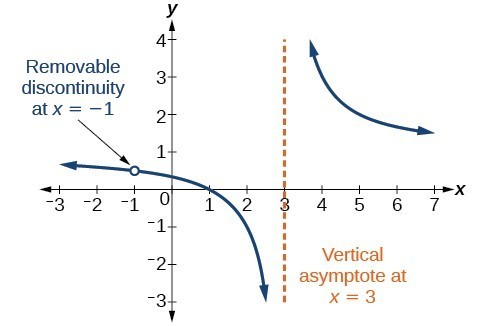### A General Note: Removable Discontinuities of Rational Functions

A removable discontinuity occurs in the graph of a rational function at $x=a$ if a is a zero for a factor in the denominator that is common with a factor in the numerator. We factor the numerator and denominator and check for common factors. If we find any, we set the common factor equal to 0 and solve. This is the location of the removable discontinuity. This is true if the multiplicity of this factor is greater than or equal to that in the denominator. If the multiplicity of this factor is greater in the denominator, then there is still an asymptote at that value.

### Example: Identifying Vertical Asymptotes and Removable Discontinuities for a Graph

Find the vertical asymptotes and removable discontinuities of the graph of $k\left(x\right)=\dfrac{x - 2}{{x}^{2}-4}$.

### Try It

Find the vertical asymptotes and removable discontinuities of the graph of $f\left(x\right)=\dfrac{{x}^{2}-25}{{x}^{3}-6{x}^{2}+5x}$.

## Horizontal Asymptotes and Intercepts

While vertical asymptotes describe the behavior of a graph as the output gets very large or very small, horizontal asymptotes help describe the behavior of a graph as the input gets very large or very small. Recall that a polynomial’s end behavior will mirror that of the leading term. Likewise, a rational function’s end behavior will mirror that of the ratio of the leading terms of the numerator and denominator functions.

There are three distinct outcomes when checking for horizontal asymptotes:

Case 1: If the degree of the denominator > degree of the numerator, there is a horizontal asymptote at $y=0$.

Example: $f\left(x\right)=\dfrac{4x+2}{{x}^{2}+4x - 5}$

In this case the end behavior is $f\left(x\right)\approx \frac{4x}{{x}^{2}}=\frac{4}{x}$. This tells us that, as the inputs increase or decrease without bound, this function will behave similarly to the function $g\left(x\right)=\frac{4}{x}$, and the outputs will approach zero, resulting in a horizontal asymptote at $y=0$. Note that this graph crosses the horizontal asymptote.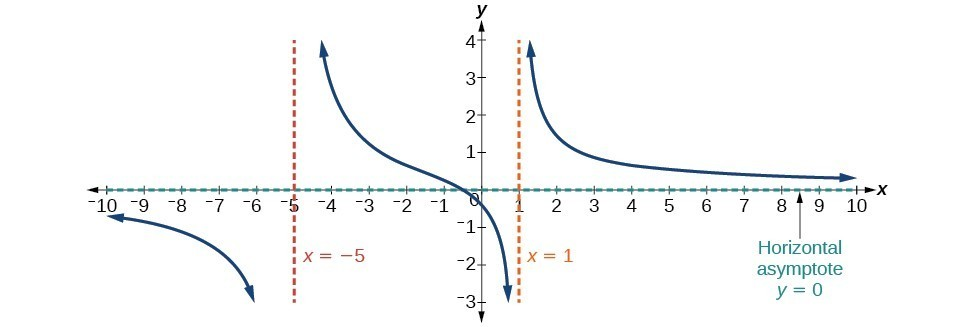Horizontal Asymptote $y=0$ when $f\left(x\right)=\dfrac{p\left(x\right)}{q\left(x\right)},q\left(x\right)\ne{0}\text{ where degree of }p<\text{degree of q}$.

Case 2: If the degree of the denominator < degree of the numerator by one, we get a slant asymptote.

Example: $f\left(x\right)=\dfrac{3{x}^{2}-2x+1}{x - 1}$

In this case the end behavior is $f\left(x\right)\approx \frac{3{x}^{2}}{x}=3x$. This tells us that as the inputs increase or decrease without bound, this function will behave similarly to the function $g\left(x\right)=3x$. As the inputs grow large, the outputs will grow and not level off, so this graph has no horizontal asymptote. However, the graph of $g\left(x\right)=3x$ looks like a diagonal line, and since $f$ will behave similarly to $g$, it will approach a line close to $y=3x$. This line is a slant asymptote.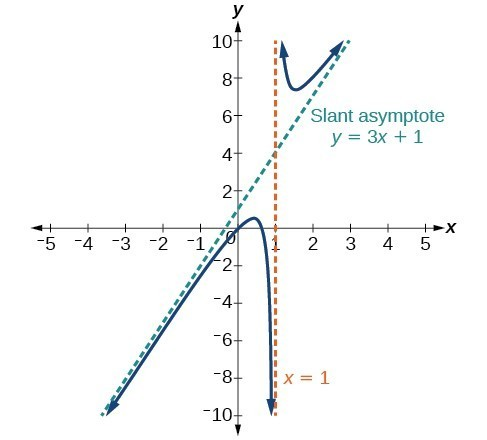Slant Asymptote when $f\left(x\right)=\dfrac{p\left(x\right)}{q\left(x\right)},q\left(x\right)\ne 0$ where degree of $p>\text{ degree of }q\text{ by }1$.

To find the equation of the slant asymptote, divide $\dfrac{3{x}^{2}-2x+1}{x - 1}$. The quotient is $3x+1$, and the remainder is 2. The slant asymptote is the graph of the line $g\left(x\right)=3x+1$.

Case 3: If the degree of the denominator = degree of the numerator, there is a horizontal asymptote at $y=\frac{{a}_{n}}{{b}_{n}}$, where ${a}_{n}$ and ${b}_{n}$ are the leading coefficients of $p\left(x\right)$ and $q\left(x\right)$ for $f\left(x\right)=\frac{p\left(x\right)}{q\left(x\right)},q\left(x\right)\ne 0$.

Example: $f\left(x\right)=\dfrac{3{x}^{2}+2}{{x}^{2}+4x - 5}$

In this case the end behavior is $f\left(x\right)\approx \frac{3{x}^{2}}{{x}^{2}}=3$. This tells us that as the inputs grow large, this function will behave like the function $g\left(x\right)=3$, which is a horizontal line. As $x\to \pm \infty ,f\left(x\right)\to 3$, resulting in a horizontal asymptote at $y=3$. Note that this graph crosses the horizontal asymptote.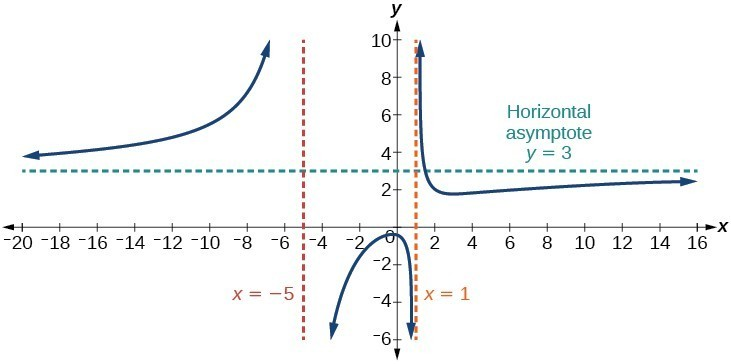Horizontal Asymptote when $f\left(x\right)=\frac{p\left(x\right)}{q\left(x\right)},q\left(x\right)\ne 0\text{ where degree of }p=\text{degree of }q$.

Notice that, while the graph of a rational function will never cross a vertical asymptote, the graph may or may not cross a horizontal or slant asymptote. Also, although the graph of a rational function may have many vertical asymptotes, the graph will have at most one horizontal (or slant) asymptote.

It should be noted that, if the degree of the numerator is larger than the degree of the denominator by more than one, the end behavior of the graph will mimic the behavior of the reduced end behavior fraction. For instance, if we had the function

$f\left(x\right)=\dfrac{3{x}^{5}-{x}^{2}}{x+3}$

with end behavior

$f\left(x\right)\approx \dfrac{3{x}^{5}}{x}=3{x}^{4}$,

the end behavior of the graph would look similar to that of an even polynomial with a positive leading coefficient.

As $x\to \pm \infty , f\left(x\right)\to \infty$

### A General Note: Horizontal Asymptotes of Rational Functions

The horizontal asymptote of a rational function can be determined by looking at the degrees of the numerator and denominator.

• Degree of numerator is less than degree of denominator: horizontal asymptote at $y=0$.
• Degree of numerator is greater than degree of denominator by one: no horizontal asymptote; slant asymptote.
• Degree of numerator is equal to degree of denominator: horizontal asymptote at ratio of leading coefficients.

### Example: Identifying Horizontal and Slant Asymptotes

For the functions below, identify the horizontal or slant asymptote.

1. $g\left(x\right)=\dfrac{6{x}^{3}-10x}{2{x}^{3}+5{x}^{2}}$
2. $h\left(x\right)=\dfrac{{x}^{2}-4x+1}{x+2}$
3. $k\left(x\right)=\dfrac{{x}^{2}+4x}{{x}^{3}-8}$

### Try it

Watch this video to see more worked examples of determining which kind of horizontal asymptote a rational function will have.

### Example: Identifying Horizontal Asymptotes

In the sugar concentration problem earlier, we created the equation $C\left(t\right)=\dfrac{5+t}{100+10t}$.

Find the horizontal asymptote and interpret it in context of the problem.

### Example: Identifying Horizontal and Vertical Asymptotes

Find the horizontal and vertical asymptotes of the function

$f\left(x\right)=\dfrac{\left(x - 2\right)\left(x+3\right)}{\left(x - 1\right)\left(x+2\right)\left(x - 5\right)}$

### Try It

Find the vertical and horizontal asymptotes of the function:

$f\left(x\right)=\frac{\left(2x - 1\right)\left(2x+1\right)}{\left(x - 2\right)\left(x+3\right)}$

### A General Note: Intercepts of Rational Functions

A rational function will have a y-intercept when the input is zero, if the function is defined at zero. A rational function will not have a $y$-intercept if the function is not defined at zero.

Likewise, a rational function will have $x$-intercepts at the inputs that cause the output to be zero. Since a fraction is only equal to zero when the numerator is zero, $x$-intercepts can only occur when the numerator of the rational function is equal to zero.

### Example: Finding the Intercepts of a Rational Function

Find the intercepts of $f\left(x\right)=\dfrac{\left(x - 2\right)\left(x+3\right)}{\left(x - 1\right)\left(x+2\right)\left(x - 5\right)}$.

### Try It

Given the reciprocal squared function that is shifted right 3 units and down 4 units, write this as a rational function. Then, find the $x$– and $y$-intercepts and the horizontal and vertical asymptotes.

Watch the following video to see more worked examples of finding asymptotes, intercepts and holes of rational functions.

## Graph Rational Functions

Previously we saw that the numerator of a rational function reveals the $x$-intercepts of the graph, whereas the denominator reveals the vertical asymptotes of the graph. As with polynomials, factors of the numerator may have integer powers greater than one. Fortunately, the effect on the shape of the graph at those intercepts is the same as we saw with polynomials.

The vertical asymptotes associated with the factors of the denominator will mirror one of the two toolkit reciprocal functions. When the degree of the factor in the denominator is odd, the distinguishing characteristic is that on one side of the vertical asymptote the graph heads towards positive infinity, and on the other side the graph heads towards negative infinity.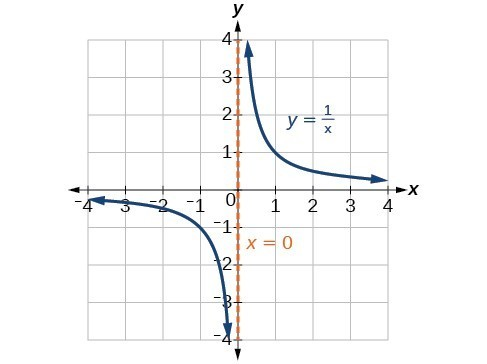When the degree of the factor in the denominator is even, the distinguishing characteristic is that the graph either heads toward positive infinity on both sides of the vertical asymptote or heads toward negative infinity on both sides.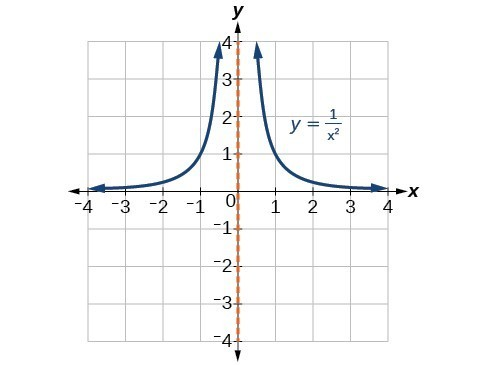For example the graph of $f\left(x\right)=\dfrac{{\left(x+1\right)}^{2}\left(x - 3\right)}{{\left(x+3\right)}^{2}\left(x - 2\right)}$.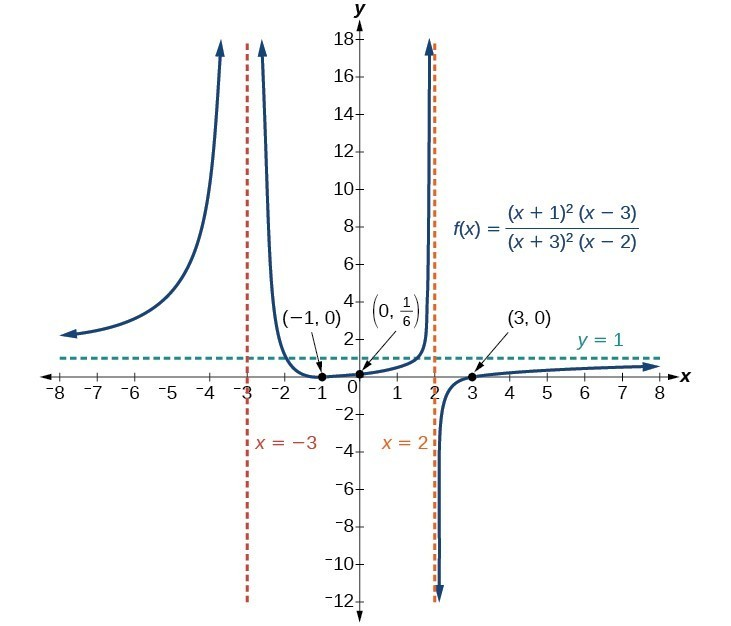• At the $x$-intercept $x=-1$ corresponding to the ${\left(x+1\right)}^{2}$ factor of the numerator, the graph bounces, consistent with the quadratic nature of the factor.
• At the $x$-intercept $x=3$ corresponding to the $\left(x - 3\right)$ factor of the numerator, the graph passes through the axis as we would expect from a linear factor.
• At the vertical asymptote $x=-3$ corresponding to the ${\left(x+3\right)}^{2}$ factor of the denominator, the graph heads towards positive infinity on both sides of the asymptote, consistent with the behavior of the function $f\left(x\right)=\frac{1}{{x}^{2}}$.
• At the vertical asymptote $x=2$, corresponding to the $\left(x - 2\right)$ factor of the denominator, the graph heads towards positive infinity on the left side of the asymptote and towards negative infinity on the right side, consistent with the behavior of the function $f\left(x\right)=\frac{1}{x}$.

### How To: Given a rational function, sketch a graph.

1. Evaluate the function at 0 to find the $y$-intercept.
2. Factor the numerator and denominator.
3. For factors in the numerator not common to the denominator, determine where each factor of the numerator is zero to find the $x$-intercepts.
4. Find the multiplicities of the $x$-intercepts to determine the behavior of the graph at those points.
5. For factors in the denominator, note the multiplicities of the zeros to determine the local behavior. For those factors not common to the numerator, find the vertical asymptotes by setting those factors equal to zero and then solve.
6. For factors in the denominator common to factors in the numerator, find the removable discontinuities by setting those factors equal to 0 and then solve.
7. Compare the degrees of the numerator and the denominator to determine the horizontal or slant asymptotes.
8. Sketch the graph.

### Example: Graphing a Rational Function

Sketch a graph of $f\left(x\right)=\dfrac{\left(x+2\right)\left(x - 3\right)}{{\left(x+1\right)}^{2}\left(x - 2\right)}$.

### Try It

Given the function $f\left(x\right)=\dfrac{{\left(x+2\right)}^{2}\left(x - 2\right)}{2{\left(x - 1\right)}^{2}\left(x - 3\right)}$, use the characteristics of polynomials and rational functions to describe its behavior and sketch the function.

Watch the following video to see another worked example of how to match different kinds of rational functions with their graphs.

## Writing Rational Functions

Now that we have analyzed the equations for rational functions and how they relate to a graph of the function, we can use information given by a graph to write the function. A rational function written in factored form will have an $x$-intercept where each factor of the numerator is equal to zero. (An exception occurs in the case of a removable discontinuity.) As a result, we can form a numerator of a function whose graph will pass through a set of $x$-intercepts by introducing a corresponding set of factors. Likewise, because the function will have a vertical asymptote where each factor of the denominator is equal to zero, we can form a denominator that will produce the vertical asymptotes by introducing a corresponding set of factors.

### A General Note: Writing Rational Functions from Intercepts and Asymptotes

If a rational function has $x$-intercepts at $x={x}_{1}, {x}_{2}, …, {x}_{n}$, vertical asymptotes at $x={v}_{1},{v}_{2},\dots ,{v}_{m}$, and no ${x}_{i}=\text{any }{v}_{j}$, then the function can be written in the form:

$f\left(x\right)=a\frac{{\left(x-{x}_{1}\right)}^{{p}_{1}}{\left(x-{x}_{2}\right)}^{{p}_{2}}\cdots {\left(x-{x}_{n}\right)}^{{p}_{n}}}{{\left(x-{v}_{1}\right)}^{{q}_{1}}{\left(x-{v}_{2}\right)}^{{q}_{2}}\cdots {\left(x-{v}_{m}\right)}^{{q}_{n}}}$

where the powers ${p}_{i}$ or ${q}_{i}$ on each factor can be determined by the behavior of the graph at the corresponding intercept or asymptote, and the stretch factor $a$ can be determined given a value of the function other than the $x$-intercept or by the horizontal asymptote if it is nonzero.

### How To: Given a graph of a rational function, write the function.

1. Determine the factors of the numerator. Examine the behavior of the graph at the x-intercepts to determine the zeroes and their multiplicities. (This is easy to do when finding the “simplest” function with small multiplicities—such as 1 or 3—but may be difficult for larger multiplicities—such as 5 or 7, for example.)
2. Determine the factors of the denominator. Examine the behavior on both sides of each vertical asymptote to determine the factors and their powers.
3. Use any clear point on the graph to find the stretch factor.

### Example: Writing a Rational Function from Intercepts and Asymptotes

Write an equation for the rational function below.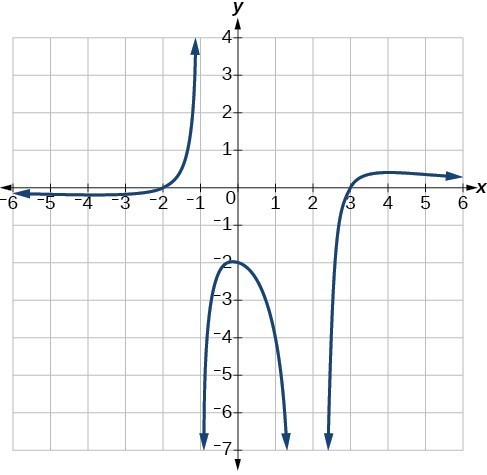## Key Equations

 Rational Function $f\left(x\right)=\dfrac{P\left(x\right)}{Q\left(x\right)}=\dfrac{{a}_{p}{x}^{p}+{a}_{p - 1}{x}^{p - 1}+…+{a}_{1}x+{a}_{0}}{{b}_{q}{x}^{q}+{b}_{q - 1}{x}^{q - 1}+…+{b}_{1}x+{b}_{0}}, Q\left(x\right)\ne 0$

## Key Concepts

• We can use arrow notation to describe local behavior and end behavior of the toolkit functions $f\left(x\right)=\frac{1}{x}$ and $f\left(x\right)=\frac{1}{{x}^{2}}$.
• A function that levels off at a horizontal value has a horizontal asymptote. A function can have more than one vertical asymptote.
• Application problems involving rates and concentrations often involve rational functions.
• The domain of a rational function includes all real numbers except those that cause the denominator to equal zero.
• The vertical asymptotes of a rational function will occur where the denominator of the function is equal to zero and the numerator is not zero.
• A removable discontinuity might occur in the graph of a rational function if an input causes both numerator and denominator to be zero.
• A rational function’s end behavior will mirror that of the ratio of the leading terms of the numerator and denominator functions.
• Graph rational functions by finding the intercepts, behavior at the intercepts and asymptotes, and end behavior.
• If a rational function has x-intercepts at $x={x}_{1},{x}_{2},\dots ,{x}_{n}$, vertical asymptotes at $x={v}_{1},{v}_{2},\dots ,{v}_{m}$, and no ${x}_{i}=\text{any }{v}_{j}$, then the function can be written in the form $f\left(x\right)=a\dfrac{{\left(x-{x}_{1}\right)}^{{p}_{1}}{\left(x-{x}_{2}\right)}^{{p}_{2}}\cdots {\left(x-{x}_{n}\right)}^{{p}_{n}}}{{\left(x-{v}_{1}\right)}^{{q}_{1}}{\left(x-{v}_{2}\right)}^{{q}_{2}}\cdots {\left(x-{v}_{m}\right)}^{{q}_{n}}}$

## Glossary

arrow notation
a way to symbolically represent the local and end behavior of a function by using arrows to indicate that an input or output approaches a value
horizontal asymptote
a horizontal line $y=b$ where the graph approaches the line as the inputs increase or decrease without bound.
rational function
a function that can be written as the ratio of two polynomials
removable discontinuity
a single point at which a function is undefined that, if filled in, would make the function continuous; it appears as a hole on the graph of a function
vertical asymptote
a vertical line $x=a$ where the graph tends toward positive or negative infinity as the inputs approach $a$